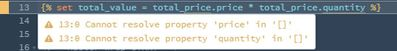## CMS DevelopmentContributeur de premier rang

SOLVE

Hello, does anyone know how I can add the contents of a column pulled from HubDB?

I have a column called 'price' and I want to add up all the rows that are still available for purchase for example:

``````{% set price = hubdb_table_rows(1234567) %}

{% set total_price = price | rejectattr ("price","equalto",blank) | selectattr ("status","equalto","Available") %}``````

The above is filtering the data as needed, but I just can't figure out the total value from this filter. For example each item is £10 and 4 are left to be sold. I need the total to show that the total value of items left to sell is £40.

1 Accepted solutionSolution

SOLVE

Hi @ben-duchy (and @Teun) just going to add my two pence here. It looks like 'total_price' is a list of rows from your HubDB, so you need to loop over them and add the value of the price column to a variable set outside of said loop. Something like this:

``````{% set price = hubdb_table_rows(1234567) %}
{% set price_rows = price | rejectattr ("price","equalto",blank) | selectattr ("status","equalto","Available") %}
{% set totalPrice = {'value': 0} %}
{% for row in price_rows %}
{# using cut and int here to make sure the value is just an integer #}
{% do totalPrice.update({'value': totalPrice.value + row.price|cut('£')|int}) %}
{% endfor %}
<p>Total value: £{{totalPrice.value}}</p>``````Contributeur de premier rang

SOLVE

That's perfect, thanks @piersgSolution

SOLVE

Hi @ben-duchy (and @Teun) just going to add my two pence here. It looks like 'total_price' is a list of rows from your HubDB, so you need to loop over them and add the value of the price column to a variable set outside of said loop. Something like this:

``````{% set price = hubdb_table_rows(1234567) %}
{% set price_rows = price | rejectattr ("price","equalto",blank) | selectattr ("status","equalto","Available") %}
{% set totalPrice = {'value': 0} %}
{% for row in price_rows %}
{# using cut and int here to make sure the value is just an integer #}
{% do totalPrice.update({'value': totalPrice.value + row.price|cut('£')|int}) %}
{% endfor %}
<p>Total value: £{{totalPrice.value}}</p>``````SOLVE

Hi @ben-duchy ,

This should be the correct answer. I misread and thought you were retrieving a single row.Contributeur de premier rang

SOLVE

My apologies, I don't think my original post was very clear.

Ignoring my example and just thinking generically for a moment, how would you filter out just that 1 column in order to add up the contents? Would the column need to be set as a number?SOLVE

Hi @ben-duchy ,

Could you help me out here, please show me the result of

``{{total_price|pprint}}``

Just so I know what we are dealing with. If the value of total_price is an object, you should be able to make a simple calculation. Something like:

``{% set total_value = total_price.price * total_price.quantity %}``Contributeur de premier rang

SOLVE

Hi @Teun,

Using the below returns (SizeLimitingPyList: []) but I'm not sure what this means.

``{{total_price|pprint}}``

When entering your example calculation (below) shows errors

``{% set total_value = total_price.price * total_price.quantity %}``